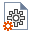Join one of our upcoming courses taking place around the world! Explore now

# Capacity Table Generator Overview

The purpose of the Capacity Table Generator feature is to calculate pile capacities at a given location for different types of piles (where for example, cross-section properties are changed), and at varying pile lengths. These results can then be output into a table and series of graphs.

Currently, only Bored piles with a single pile section (or single section with bell) is supported in the Capacity Table Generator.

## Zones

The locations are simplified into rectangular capacity Zones representing an area with averaged soil properties, interpolated at the center of the Zone. Different pile types can be investigated and computed within each Zone.

## Zone Capacity Table

Zone Capacity Tables (and graphs) can be batch generated from calculated total capacity, skin friction capacity, and end bearing capacities of these piles. Based on this information, the engineer or designer can make decisions on what pile types to use in each location.

## User-defined Factors of Safety

The table and graph results show the unfactored (i.e., ultimate) capacities calculated over the depth of each pile. The results can also show factored (i.e., allowable) capacities from user-defined factors of safety for:

• Total Capacity (defined by a single factor "FS1" for the combined effects of Skin Friction and End Bearing)
• Capacity based on Skin Friction (ignoring End Bearing) ("FS2")
• Skin Friction and End Bearing (defined by two separate factors, one for Skin Friction "FS3", and another for End Bearing "FS4")

To show allowable capacities in the Zone Capacity Table (and graphs):

1. Select Project Settingsfrom the Home tab.
2. Navigate to the Capacity Calculations tab.
3. Under Calculate Allowable Capacities:
1. Select the Total Capacity checkbox and set the FS1 value.
2. Select the Skin Friction checkbox and set the FS2 value.
3. Select the Skin Friction / End Bearing checkbox and set the FS3 and FS4 values.
4. Select OK to exit the dialog.

### Allowable Total Capacity

The Allowable Total Capacity is calculated as follows:

• Allowable Total Capacity = Ultimate Total Capacity / FS1

### Allowable Capacity based on Skin Friction Only

The Allowable Skin Friction Capacity is calculated as follows:

• Allowable Skin Friction Capacity = Ultimate Skin Friction Capacity / FS2

### Allowable Skin Friction & End Bearing Capacity

The Allowable Total Capacity is calculated as follows:

• Skin Friction & End Bearing Capacity = (Ultimate Skin Friction Capacity / FS3) + (Ultimate End Bearing Capacity / FS4)

## Limit Average Stress

In some cases, you may want to flag some calculated values in the tables if they exceed the average stress criteria. If Limit Average Stress is selected in Project Settings, any allowable capacity value exceeding the computed average allowable load carrying capacity of a pile will be highlighted in RED.

The Average Allowable Load Carrying Capacity of a Pile is calculated as follows:

• Average Allowable Load Carrying Capacity of a Pile = (0.25 x Concrete Cylinder Strength) / 0.8 x Cross-sectional Area of Pile